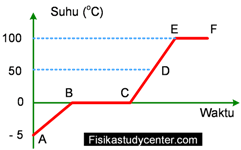# Fisika Study Center

## 9 Common Problems of Heat for Junior

physics.fisikastudycenter.com - Learning heat for junior hingh school students. Given 9 common problems includes the solutions, the specific heat, the heat of fusion and black principle in heat exchange will be discussed.Problem 1
Water with a mass of 100 gram at a temperature of 20° C then heated to a temperature of 80° C. If the apecific heat of water is 1 cal/gr ° C what is the heat needed in this process show your answer in calorie!

Answer
Datal:
m = 100 gram
c = 1 cal/gr°C
T1 = 20°C
T2 = 80°C

The heat needed:
Q = m x c x Δ T
Q = 100 x 1 x (80−20)
Q = 100 x 60
Q = 6000 calorie

Problem 2
100 gram of water at a temperature of 20° C is heated to the temperature of 100°. If the specific heat of water is 4200 J/kg ° C find the heat taken in this process, show in joule!

Answer
m = 100 gram = 0.1 kg
c = 4200 J/kg °C
T1 = 20°C
T2 = 100°C
The heat needed:
Q = m x c x Δ T
Q = 0.1 x 4200 x (100−20)
Q = 420 x 80
Q = 33600 joule

Problem 3
200 gram of ice at − 5°C is heated to a temperature of − 1° C, if the specific heat of ice is 0.5 kal/gr ° C determine the heat taken in this process!

Answer
m = 200 gram
c = 0.5 kal/gr°C
T1 = −5°C
T2 = −1°C

The heat:
Q = m x c x Δ T
Q = 200 x 0.5 x [−1−(−5)]
Q = 100 x 4
Q = 400 kalori

Problem 4
What is heat needed to melt 150 gram of ice to liquid water of 0°C, use the heat of fusion of ice is 80 cal/g!

Answer
Data :
m = 150 gram
L = 80 kal/gr
Heat to melt the ice:
Q = m x L
Q = 150 x 80
Q = 12000 calorie

Problem 5
250 gram of ice at a temperature of − 5° C is heated and melts to liquid water of 0°C. If the specific heat of ice is 0.5 cal/gr°C, and the heat of fusion of ice is 80 cal/gr, find the heat taken!

Answer
Data:
m = 250 gram
ces = 0.5 kal/gr°C
Les = 80 cal/gram

Step 1, raising the temperature of the ice:
Q1 = m x c x ΔT
Q1 = 250 x 0,5 x 5
Q1 = 625 calorie

Step 2, melting the ice:
Q2 = m x L = 250 x 80 = 20000 kalori

The total heat Q1 + Q2
Q = 625 + 20000
Q = 20625 calorie

Problem 6
200 gram of ice at − 5° C is heated to a liquid water of 100°C. If the specific heat of ice is 0.5 cal/gr°C, the specific heat of water is 1 cal/gr°C and the heat of fusion of ice is 80 cal/gr, find the heat needed in the process!

Answer
Datal:
mes = mair = 200 gram
ces = 0.5 cal/gr°C
cair = 1 cal/gr°C
Les = 80 cal/gram

Step 1, raisng ice temperature :
Q1 = m x c x ΔT
Q1 = 200 x 0.5 x 5
Q1 = 500 calorie

Step 2, melting ice:
Q2 = m x L = 200 x 80 = 16000 calorie

Step 3, raising the water temperature from 0oC to 100o C
Q3 = m x c x ΔT
Q3 = 200 x 1 x 100
Q3 = 30000 calorie

The total heat Q1, Q2 dan Q3:
Q = 500 + 16000 + 30000
Q = 46500 calorie

Problem 7
200 gram of water at 20°C is mixed with 300 gram of water at 90°C, calculate the mixture temperature!

Answer
Data :
m1 = 200 gram
m2 = 300 gram
c1 = c2 = 1 cal/gr°C
ΔT1 = t − 20
ΔT2 = 90 − t

Black principle of heat exchange:
m2 x c2 x ΔT2 = m1 x c1 x ΔT1
300 x 1 x (90 − t) = 200 x 1 x (t − 20)
27000 − 300t = 200t − 4000
27000 + 4000 = 300t + 200t
31000 = 500t
t = 31000 / 500
t = 62°C

Problem 8
metal at 30°C  of specific heat of 0,2 kal/gr°C with 100 gram of mass is dropped into water of 200 gram of mass at a temperature of 90°C. If the specific heat of water is 1 ckal/gr°C calculate the final temperature of the metal, ignore the heat lost in surrounding!

Answer
Datal:
m1 = 100 gram
m2 = 200 gram
c1 = 0.2 cal/gr°
c2 = 1 cal/gr°C
ΔT1 = t − 30
ΔT2 = 90 − t

Black principle
m2 x c2 x ΔT2 = m1 x c1 x ΔT1
200 x 1 x (90 − t) = 100 x 0,2 x (t − 30)
18000 − 200t = 20 t − 600
18000 + 600 = 200t + 20t
18600 = 220t
t = 18600 / 220
t = 84,5 °C

Problem 9
Given a graph below the temperature vs time:500 gram of water raises its temperature from C to D. If the specific heat of water is 4200 J/kg°C, find the heat needed in the process, show your answer in kilojoule!

Answer
Q = m x c x Δ T
Q = 0.5 x 4200 x 50
Q = 105000 joule
Q = 105 kJ

prep. by:
physics.fisikastudycenter.com

Joomla Templates: from JoomlaShack.com
Template Upgrade by Joomla Visually